# Introduction to Mensuration

• Difficulty Level : Basic
• Last Updated : 24 Dec, 2021

Mensuration is the branch of geometry that deals with the measurement of area, length, or volume in 2D and 3D shapes. The 2D shapes can be drawn in a plane like square, rectangle, triangle, circle, etc. and 3D shapes cannot be represented in a plane like bricks, ice-cream cones, football, etc. Mensuration includes computation using mathematical formulas and algebraic equations.

## Types of Geometrical Shapes –

2-Dimensional Shapes:

In 2D objects having only two dimensions, such as width and height but no thickness. Like a square, rectangle, triangle, circle. In mathematical representation, it has Two-axis (X and Y). Having only two-axis and no thickness, these 2D objects do not exist in the real world and can be represented only by using plain surfaces.

Example: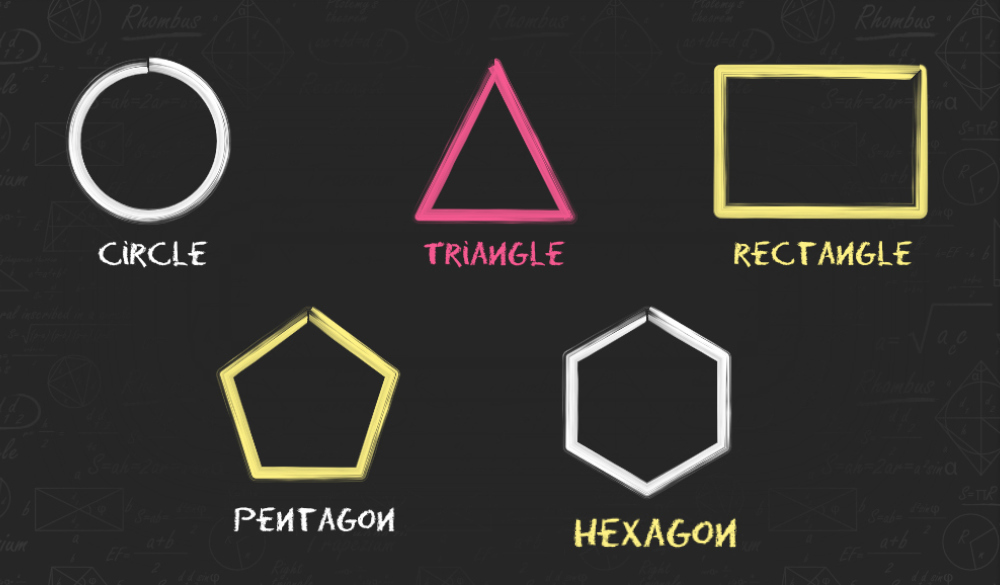3-Dimensional Shapes:

In 3D object having three dimensions (such as height, width, and depth), like any object in the real world. In mathematical representation, it has three-axis (X, Y, and Z). Unlike 2D shapes, 3D shapes have more parameters to cover. 3D objects have some volume and Total Surface area that uses all the three dimensions i.e. length, width, and depth of the object.

Example: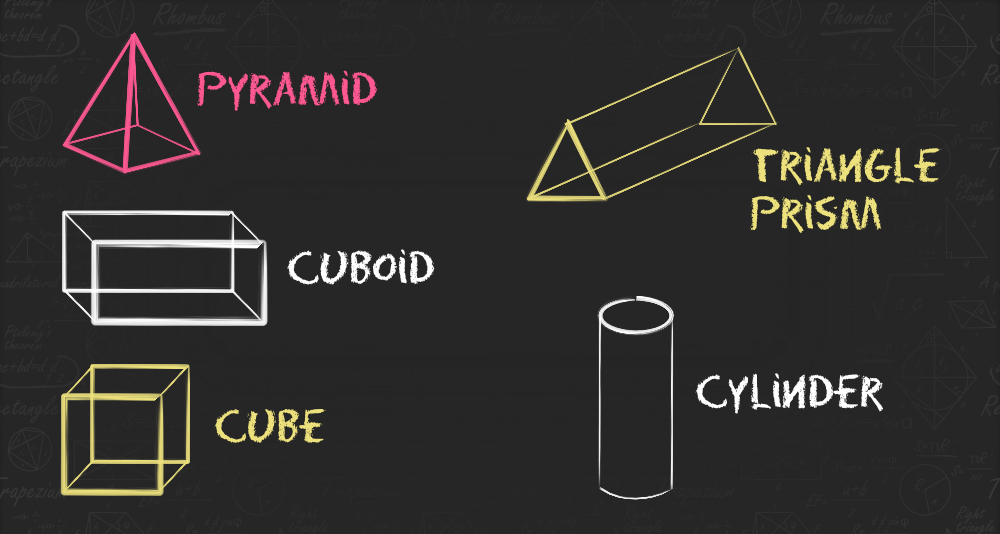## Formulas for 2D shapes –

A. Rectangle:

A rectangle is a 2D shape, having 4 sides and 4 corners. The rectangle is a quadrilateral with four right angles, so, each angle is 90°. The sum of all the interior angles is equal to 360 degrees. The opposite sides are parallel and equal to each other. Diagonals of a rectangle have the same length.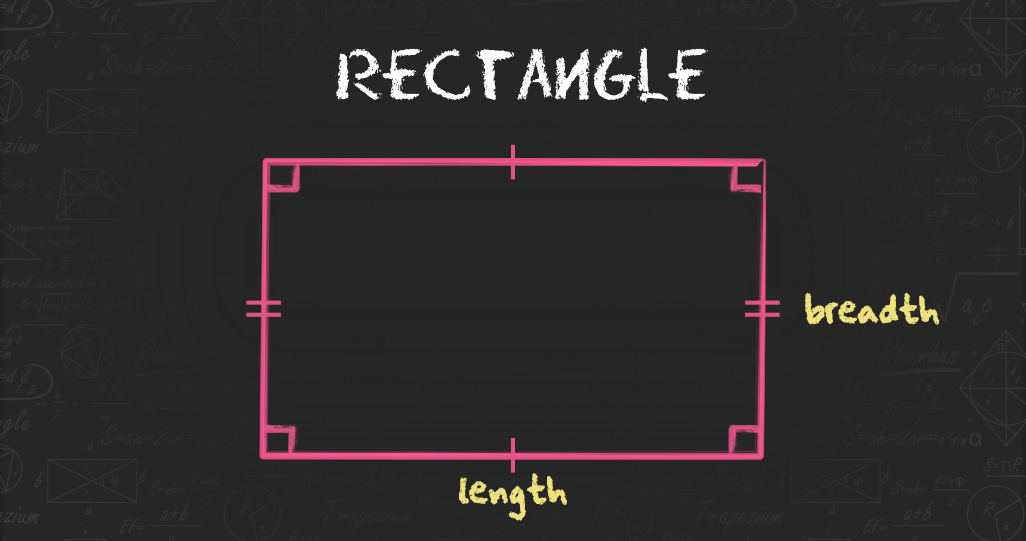Perimeter of a Rectangle = 2(Length+Breadth)

Area of a Rectangle = Length×Breadth

B. Square:

A square is a 2D shape plane figure with four equal sides and all the four angles are equal to 90 degrees. Diagonals of a square are of equal length.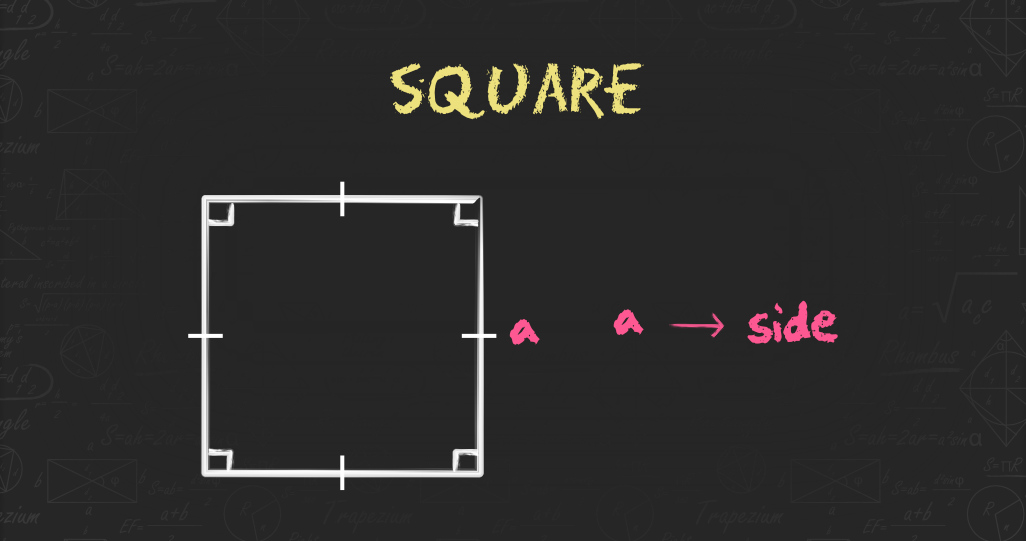Area of a Square= Side2

Perimeter of a Square= 4(Side)

C. Circle:

A circle is a basic 2D shape, and it is a set of points in a plane that are equidistant from the centre. The distance between the centre and any point on the circumference is called the radius.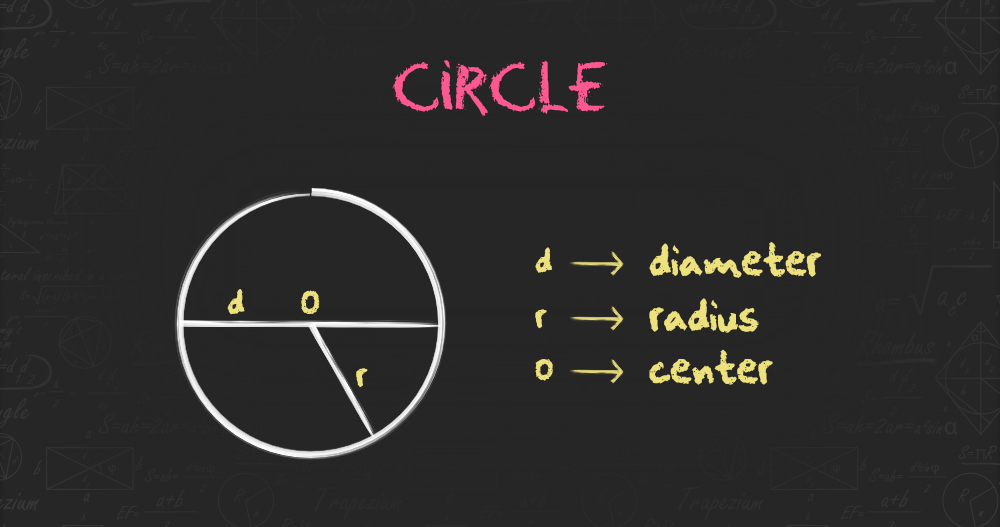Diameter of a Circle = 2 × Radius

Circumference of a Circle = π × Diameter or 2 × π × Radius

Area of a Circle = π × Radius2

D. Triangle:

A triangle has three sides and three inclusive angles. All the three angles of a triangle always add up to 180°.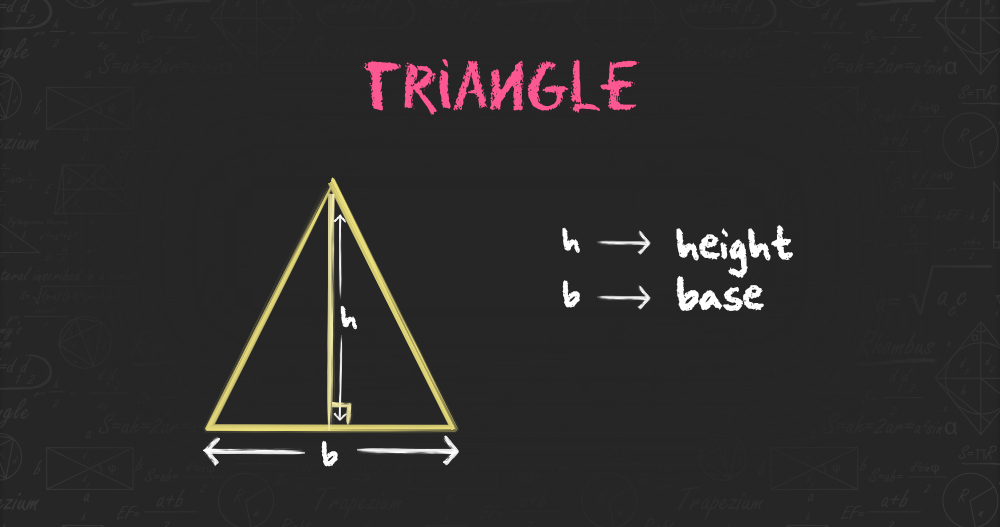Area of a Triangle = ½ × b × h

E. Parallelogram:

A parallelogram is a 2D shape whose opposite sides are parallel to each other, It has four sides, where the pair of parallel sides are equal in length.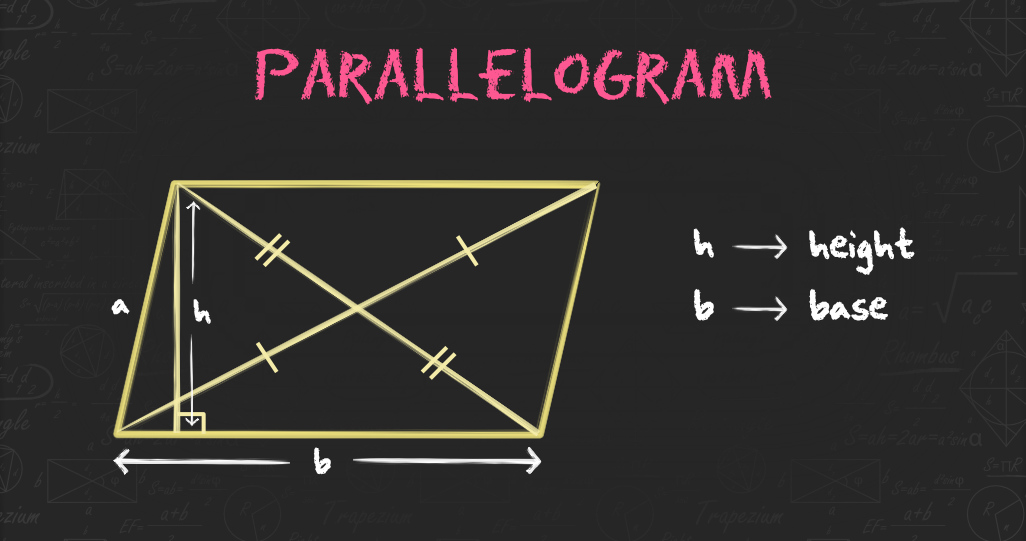Perimeter of a Parallelogram = 2 (a+b)

Area of a Parallelogram = b × h

## Formulas for 3D Shapes –

A. Cube:

Cube is a solid 3D figure, which has 6 square faces, 8 vertices, and 12 edges, such that 3 edges meet at one vertex point. An example of a cube is a piece of Sugar, ice with six square sides.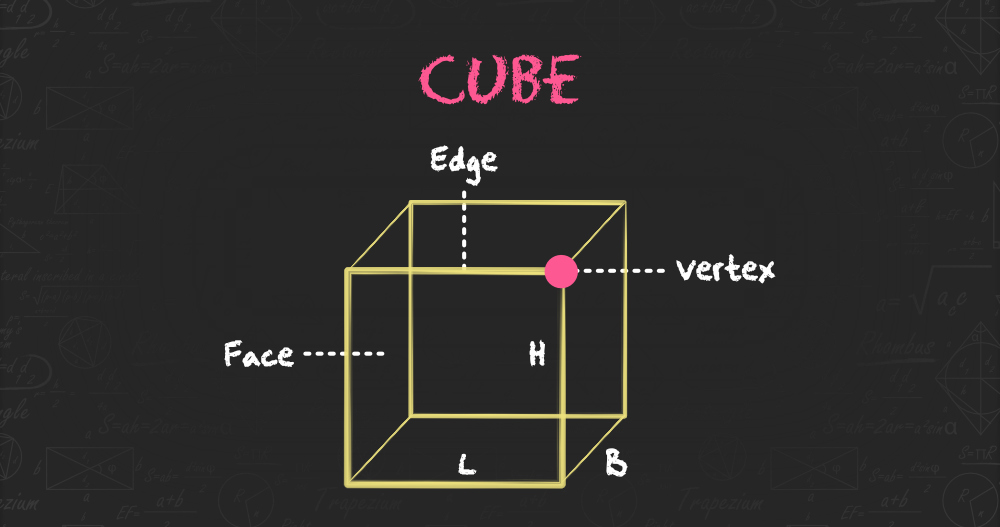Volume of a Cube = Side3 cubic units.

Lateral Surface Area of a Cube= 4 × side2 sq.units.

Total Surface Area of a Cube= 6× side2 sq. units.

B. Cuboid:

A cuboid is a 3D figure with three sides where all the sides are not equal. All of its faces are rectangles having a total of 6 faces, 8 vertices, and 12 edges.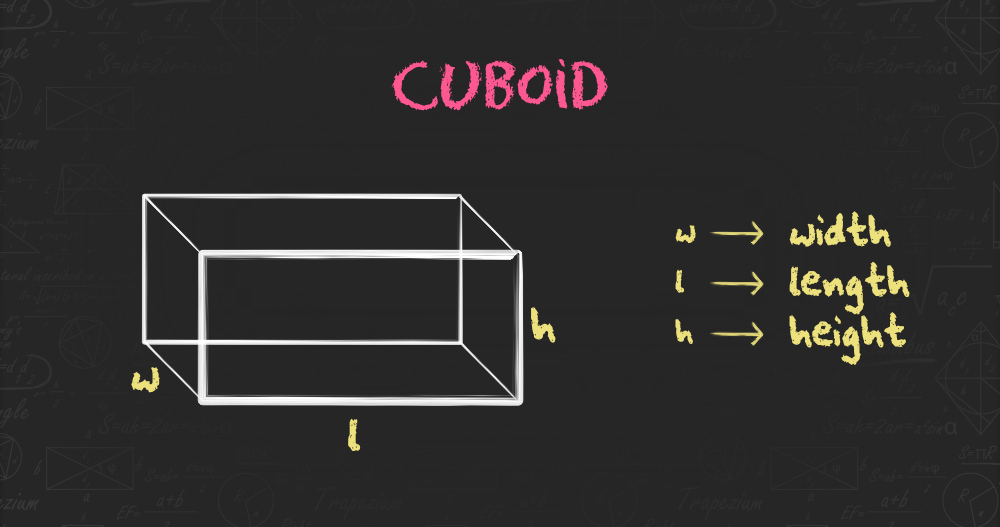Volume of a Cuboid = (length+width+height) cubic units.

Lateral Surface Area of a Cuboid = 2×height (length + width) sq. units.

Total Surface Area of a Cuboid = 2(length × width + length × height + height × width) sq.units.

Diagonal length of a Cuboid = length2 + breadth2 + height2 units.

C. Sphere:

A sphere is an object that is an absolutely round geometrical shape in 3D space. It is the set of all points in a space equidistant from a given point called the centre of the sphere. The distance between any point of the sphere and its centre is called the radius(R).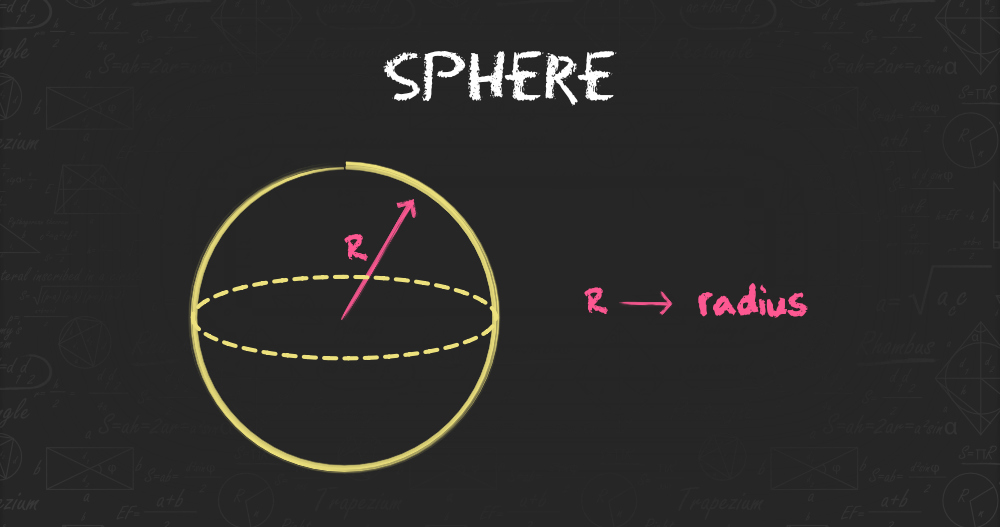Volume of a Sphere = 4/3 x π x radius³ cubic units.

Surface Area of a Sphere = 4x π x radius² sq. units.

D. Cone:

A cone is a three-dimensional geometric shape. It formed by using a set of line segments or the lines which connect a common point, called the apex or vertex. At the base of a cone it has circular, so we can compute the value of radius. And the length of the cone from apex to any point on the circumference of the base is the slant height.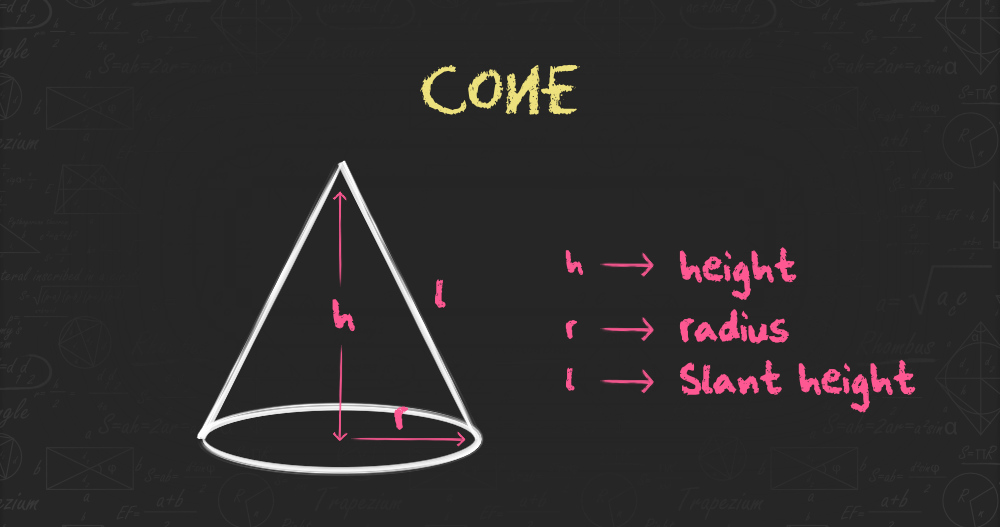Volume of a Cone = 1/3 × × π × radius² × height cubic units.

Total Surface Area of the Cone = πr(l + radius)

My Personal Notes arrow_drop_up
Recommended Articles
Page :